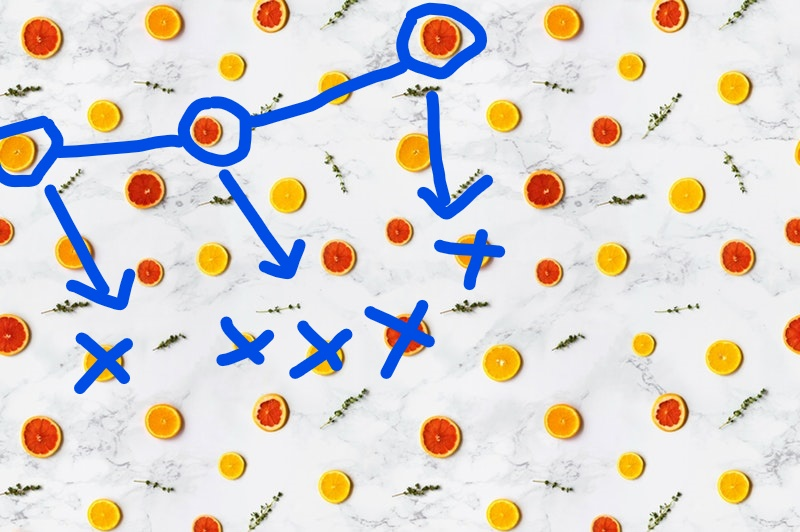Python Slice Assignment

Slice assignment is a little-used (but beautiful) Python feature that helps you replace a subsequence of a sequence.

One of my Finxter users, Mike, asked the following great question:

“I was going through a lot of slicing puzzles on the Finxter site, I came across this:

letters = ['a', 'b', 'c', 'd', 'e', 'f', 'g']
letters[1:] = [ ]
print(letters)

I originally answered [‘b’, ‘c’, ‘d’, ‘e’, ‘f’, ‘g’] but got it wrong.”

This is the point where I want to open your knowledge gap: what’s the output of this puzzle instead?

“As the answer is [‘a’], I immediately became curious because that’s [not] the answer if we use this code

letters = ['a', 'b', 'c', 'd', 'e', 'f', 'g']
print(letters[1:])
# ['b', 'c', 'd', 'e', 'f', 'g']

Why do these work differently?
Thanks, I really appreciate your time and all of the content you provide each day.”

Again, great question. Mike did most of the heavy lifting himself. The answer is simple (if you have read my slicing booklet already):

• The first version is slice assignment.
• The second version is basic slicing.

They are not the same. You should not confuse slicing and slice assignment. Here is the difference:

1) Slicing creates a new subsequence of the original sequence. You can assign this new sequence to a variable y. In essence, you overwrite any previous value of variable y:

x = list("coffeebreak")
y = list("python")

y = x[0:4]
print(''.join(y))
# 'coff'

2) Slice assignment replaces the selected slice in the original sequence y with the value specified on the right-hand side of the equation:

x = list("coffeebreak")
y = list("python")

y[0:2] = x
print(''.join(y))
# 'coffeebreakthon'

Note that the two code snippets also demonstrate how you can convert a string to a list and convert a list back to a string.

This Python lesson is based on my free “Coffee Break Python” Email Series. Join us. It’s fun! 🐍